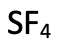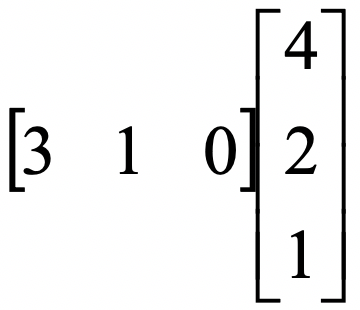# 3.5: Homework Problems Chapter 2

$$\newcommand{\vecs}{\overset { \rightharpoonup} {\mathbf{#1}} }$$ $$\newcommand{\vecd}{\overset{-\!-\!\rightharpoonup}{\vphantom{a}\smash {#1}}}$$$$\newcommand{\id}{\mathrm{id}}$$ $$\newcommand{\Span}{\mathrm{span}}$$ $$\newcommand{\kernel}{\mathrm{null}\,}$$ $$\newcommand{\range}{\mathrm{range}\,}$$ $$\newcommand{\RealPart}{\mathrm{Re}}$$ $$\newcommand{\ImaginaryPart}{\mathrm{Im}}$$ $$\newcommand{\Argument}{\mathrm{Arg}}$$ $$\newcommand{\norm}{\| #1 \|}$$ $$\newcommand{\inner}{\langle #1, #2 \rangle}$$ $$\newcommand{\Span}{\mathrm{span}}$$ $$\newcommand{\id}{\mathrm{id}}$$ $$\newcommand{\Span}{\mathrm{span}}$$ $$\newcommand{\kernel}{\mathrm{null}\,}$$ $$\newcommand{\range}{\mathrm{range}\,}$$ $$\newcommand{\RealPart}{\mathrm{Re}}$$ $$\newcommand{\ImaginaryPart}{\mathrm{Im}}$$ $$\newcommand{\Argument}{\mathrm{Arg}}$$ $$\newcommand{\norm}{\| #1 \|}$$ $$\newcommand{\inner}{\langle #1, #2 \rangle}$$ $$\newcommand{\Span}{\mathrm{span}}$$$$\newcommand{\AA}{\unicode[.8,0]{x212B}}$$

## Homework Problems

### Section 2

Exercise 1

Determine all symmetry elements and all unique symmetry operations of the following molecules:

a)b)c)d)e) bromine tetrafluoridef) Boric acidg) Dinitrogen tetroxideh)i)j)k)l)m)a)b)c)d)e)f)g)h)i)j)k)l)m)Exercise 2

When is a molecule chiral?

a) It has no mirror planes

b) It is has no inversion center

c) It has no principal axis

d) It has no rotation reflections (improper rotations)

d) It has no rotation reflections (improper rotations)

Exercise 3

If a molecule has a principal axis Cn , and n additional C2 axes standing perpendicular to Cn then it belongs to

a) A dihedral point group

b) A rotational point group

c) A low symmetry point group

d) A high symmetry point group

a) A dihedral point group

Exercise 4

Which of the following molecules are chiral:

a) CH4

b) CHCl3

c) HCFClBr

d) HOF

e) BHFCl

c) HCFClBr

Exercise 5

Determine the point groups of the molecules at symmetry.otterbein.edu/challenge/index.html until you feel that you can determine point groups effortlessly

### Section 3

Exercise 1

Can the following matrices be multiplied and if so what is the product matrix?

a)b)c)d)a)b)c)d)Exercise 2

Determine the irreducible representations for the following orbitals in the point group D2h.

The z axis is defined as the axis of the principal C2 axis. C2’ is defined as the axis rotating around y. σv is defined as the xz plane.Exercise 3

Determine the matrix representations of the symmetry elements of the following point groups:

a) D2

Define principal C2 axis as the axis running along z. C2 runs along x.

b) C3

If we define the principal C3 axis running along the z axis:

a)b)